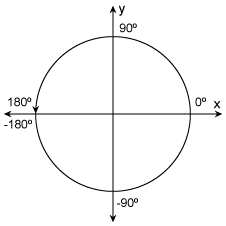# atan2d

## Syntax

``D = atan2d(Y,X)``

## Description

example

````D = atan2d(Y,X)` returns the four-quadrant inverse tangent (tan-1) of `Y` and `X`, which must be real. The result, `D`, is expressed in degrees.```

## Examples

collapse all

```x = [1 0 -1 0]; y = [0 1 0 -1]; d = atan2d(y,x)```
```d = 1×4 0 90 180 -90 ```

## Input Arguments

collapse all

y-coordinates, specified as a scalar, vector, matrix, or multidimensional array. Inputs `Y` and `X` must either be the same size or have sizes that are compatible (for example, `Y` is an `M`-by-`N` matrix and `X` is a scalar or `1`-by-`N` row vector). For more information, see Compatible Array Sizes for Basic Operations.

Data Types: `single` | `double`

x-coordinates, specified as a scalar, vector, matrix, or multidimensional array. Inputs `Y` and `X` must either be the same size or have sizes that are compatible (for example, `Y` is an `M`-by-`N` matrix and `X` is a scalar or `1`-by-`N` row vector). For more information, see Compatible Array Sizes for Basic Operations.

Data Types: `single` | `double`

collapse all

The four-quadrant inverse tangent, `atan2d(Y,X)`, returns values in the closed interval `[-180,180]` based on the values of `Y` and `X` as shown in the graphic.In contrast, `atand(Y/X)` returns results that are limited to the interval `[-90,90]`, shown on the right side of the diagram.

## Version History

Introduced in R2012b